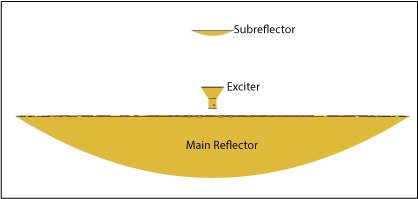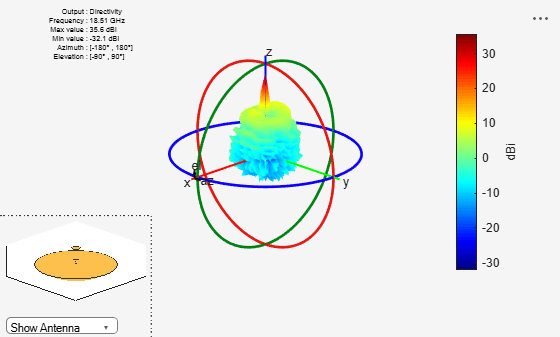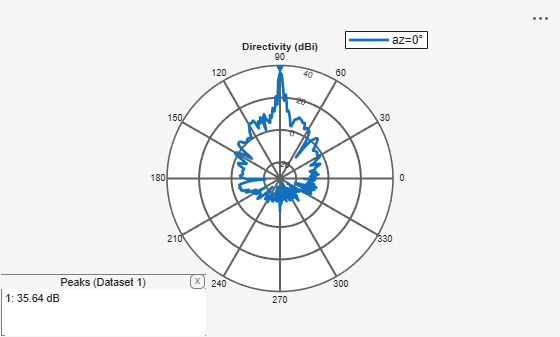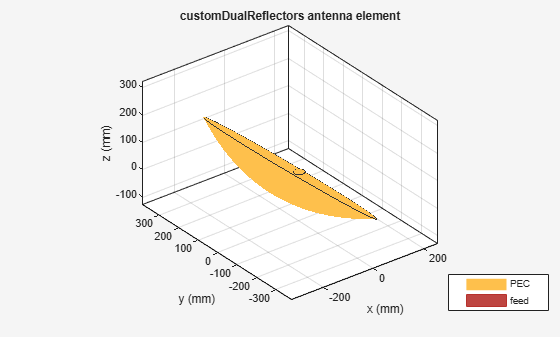# customDualReflectors

Create custom dual-reflector antenna

Since R2022a

## Description

The `customDualReflectors` object creates a dual-reflector antenna with empty geometries for the main and sub reflectors and the `hornConical` element as the default exciter. Once you create the object, you have to specify the geometry coordinates of the main and sub reflector surfaces in N-by-3 matrices and assign them to the `MainReflector` and `SubReflector` properties of the object before using the `show` function to view the antenna. N represents the total number of points to use for defining the geometry. Value of N can be different for the main and the sub reflector. Alternatively, you can also use a `triangulation` object to define the reflector geometry. You can also change the orientation of the reflectors and the exciter. You can use either a single antenna element or an array as the exciter for the object. Further, you can also create an antenna array using `customDualReflectors` object as its element. Dual-reflector antennas have very high gain and low spillover and are used in satellite communications.## Creation

### Syntax

``cdr = customDualReflectors``
``cdr = customDualReflectors(Name=Value)``

### Description

example

````cdr = customDualReflectors` creates a dual-reflector antenna with empty main and sub reflector geometries and conical horn antenna as the default exciter. After creating the object, specify the coordinates of the reflector surfaces in a N-by-3 matrix or import the coordinates from a MAT file.```

example

````cdr = customDualReflectors(Name=Value)` creates a custom dual reflector antenna, with additional Properties specified by one or more name–value arguments. `Name` is the property name and `Value` is the corresponding value. You can specify several name-value arguments in any order as `Name1`= `Value1`, `...`, `NameN`=`ValueN`. Properties not specified retain their default values. For example, `cdr = customDualReflectors(FeedOffset=[0.0850 0 0])` relocates the feed to the point (0.0850, 0, 0) with respect to the origin.```

## Properties

expand all

Exciter antenna or array, specified as an antenna or an array object, or an empty array. To create the reflector backing structure without the exciter, specify this property as an empty array.

Example: `dipole`

Example: ```dipole(Length=0.1409,Width=0.02,FeedOffset=0,Tilt=90,TiltAxis=[0 1 0])```

Example: `linearArray(Element=patchMicrostrip)`

Example: `[]`

Cartesian coordinates of the main reflector surface, specified as an N-by-3 matrix with each element unit in meters. N represents the total number of points that define the main reflector surface. You can also import the coordinates from a MAT file. This property also accepts triangulation method for defining the main reflector surface.

Example: `MainReflector=preflector`

Example: `MainReflector=triangulation(T,P)`

Data Types: `double`

Cartesian coordinates of the sub reflector surface, specified as an N-by-3 matrix with each element unit in meters. N represents the total number of points that define the sub reflector surface. You can also import the coordinates from a MAT file. This property also accepts triangulation method for defining the sub reflector surface.

Example: `SubReflector=psubreflector`

Example: `SubReflector=triangulation(T,P)`

Data Types: `double`

Cartesian coordinates of the main and sub reflector offsets with respect to the origin, specified as a 2-by-3 matrix with each element unit in meters. The first row corresponds to the main reflector offset and the second row corresponds to the sub reflector offset.

Example: `[-0.1 0 0; 0.03 0 0.224]`

Data Types: `double`

Cartesian coordinates to offset the exciter feed point, specified as a three-element vector with each element unit in meters. If you have specified an array of exciters in the `Exciter` property, use this property to offset the center of the array.

Example: `[0.0850 0 0]`

Data Types: `double`

Tilt angle of the reflectors, specified as a two-element vector with each element unit in degrees. The first element specifies the tilt of the main reflector, and the second element specifies the tilt of the sub reflector. Specify values in the range [–360, 360].

Example: `[40 200]`

Data Types: `double`

Flag to re-mesh the reflectors, specified as a numeric or logical `1(true)` or `0(false)`. Set this property to `true` to re-mesh the reflectors.

Example: `0`

Data Types: `logical` | `string`

Tilt angle of the antenna, specified as a scalar or vector with each element unit in degrees. For more information, see Rotate Antennas and Arrays.

Example: `Tilt=90`

Example: `Tilt=[90 90]`,`TiltAxis=[0 1 0;0 1 1]` tilts the antenna at 90 degrees about the two axes defined by the vectors.

Note

The `wireStack` antenna object only accepts the dot method to change its properties.

Data Types: `double`

Tilt axis of the antenna, specified as:

• Three-element vector of Cartesian coordinates in meters. In this case, each coordinate in the vector starts at the origin and lies along the specified points on the X-, Y-, and Z-axes.

• Two points in space, each specified as three-element vectors of Cartesian coordinates. In this case, the antenna rotates around the line joining the two points in space.

• A string input describing simple rotations around one of the principal axes, 'X', 'Y', or 'Z'.

Example: `TiltAxis=[0 1 0]`

Example: `TiltAxis=[0 0 0;0 1 0]`

Example: `TiltAxis = 'Z'`

Data Types: `double`

Lumped elements added to the antenna feed, specified as a `lumpedElement` object handle. You can add a load anywhere on the surface of the antenna. By default, the load is at the feed. For more information, see `lumpedElement`.

Example: `Load=lumpedElement`, where `lumpedElement` is load added to the antenna feed.

Example: `Load=lumpedElement(Impedance=75)`

Solver for antenna analysis, specified as a string. Default solver is `"MoM-PO"`(Method of Moments-Physical Optics hybrid). Other supported solvers are: `"MoM"` (Method of Moments), `"PO"` (Physical optics) or `"FMM"` (Fast Multipole Method).

Example: `SolverType`=`"MoM"`

Data Types: `string`

## Object Functions

 `show` Display antenna, array structures or shapes `impedance` Input impedance of antenna; scan impedance of array `sparameters` Calculate S-parameter for antenna and antenna array objects `returnLoss` Return loss of antenna; scan return loss of array `vswr` Voltage standing wave ratio of antenna `pattern` Radiation pattern and phase of antenna or array; Embedded pattern of antenna element in array `patternAzimuth` Azimuth pattern of antenna or array `patternElevation` Elevation pattern of antenna or array `optimize` Optimize antenna or array using SADEA optimizer `axialRatio` Axial ratio of antenna `beamwidth` Beamwidth of antenna `current` Current distribution on antenna or array surface `charge` Charge distribution on antenna or array surface `EHfields` Electric and magnetic fields of antennas; Embedded electric and magnetic fields of antenna element in arrays `mesh` Mesh properties of metal, dielectric antenna, or array structure `rcs` Calculate and plot radar cross section (RCS) of platform, antenna, or array

## Examples

collapse all

Load the MAT files containing the variables which store the coordinates for the main and sub reflector surfaces.

```load mainref.mat; %Loads a variable 'preflector' into the workspace load subref.mat; %Loads a variable 'psubreflector' into the workspace```

Create a `customDualReflectors` object by assigning the coordinates to the `MainReflector` and `SubReflector` properties.

`cdr = customDualReflectors(MainReflector=preflector,SubReflector=psubreflector)`
```cdr = customDualReflectors with properties: Exciter: [1x1 hornConical] MainReflector: [3364x3 double] SubReflector: [144x3 double] ReflectorOffset: [2x3 double] FeedOffset: [0.0064 0 0.1173] ReflectorTilt: [0 0] RemeshReflectors: 1 Tilt: 0 TiltAxis: [1 0 0] Load: [1x1 lumpedElement] SolverType: 'MoM-PO' ```

View the antenna.

`show(cdr)`Plot a 3-D radiation pattern of this antenna at 18.51 GHz.

`pattern(cdr,18.51e9) `Plot the elevation pattern of this antenna in the X-Z plane.

`pattern(cdr,18.51e9,0,1:1:360)`Tilt and offset the reflectors and the feed. View the transformed antenna.

```cdr.ReflectorTilt=[40 200]; cdr.ReflectorOffset=[-0.1 0 0;0.03 0 0.224]; cdr.FeedOffset=[0.0072 0 0.02]; show(cdr)```## Version History

Introduced in R2022a